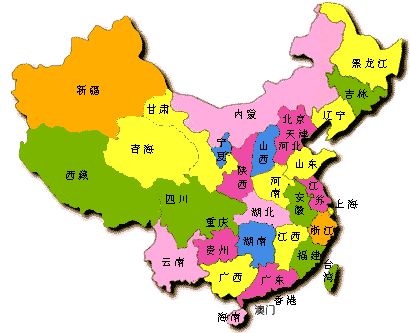﻿ ﻿ 黄博士网: 在线数学手册计算器软件，电化学虚拟实验室，虚拟电化学工作站，电化学软件 首页 | 目录 | 世界 | 学科 | 科学 | 数学 | 物理 | 化学 | 看书 | 计算 | 画图 | 编程 | 帮助 | ?++=# int 数学公式图表 dx = sum mathHandbook.com

1. 初等数学 elementary math
2. 代数 algebra
3. 函数 function
4. 几何 geometry
5. 解析几何 analytic geometry
6. 微分几何 differential geometry
7. 高等数学 higher math
8. 微积分 calculus
9. 积分变换 integral transform
10. 线性代数 linear algebra
11. 概率统计 probability
12. 改变世界面貌的十个数学公式 Great 10 formula
13. 动画 animation
14. 立体图 3D graph
15. 物理公式 Physics formula
16. 公式手册
﻿

1. 数学 - 数学符号 - 数学索引
2. 手册 = 中学数学手册 + 数学手册 + 实用数学手册
3. 初等数学 = 中学数学 = 初中数学 + 高中数学
4. 高等数学 = 基础数学 ( 代数 + 几何 + 分析 ) + 应用数学
5. 公式 - 图表 - 动画 - 立体图
6. 书单 = 数学 + 物理 + 化学 + 计算机 + 医学 + 英语 - QQ群614057790下载书
7. 数学手册计算器 = 数学 + 手册 + 计算器 + 计算机代数系统
8. 例题:
﻿